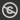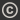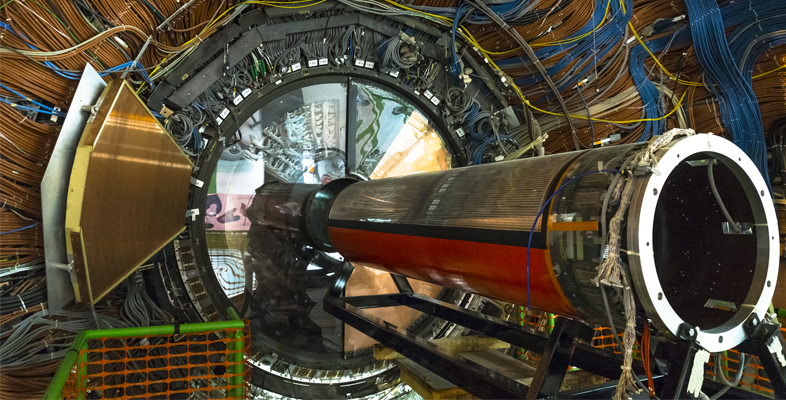## Free courses

Produced by The Open University, a world leader in open and distance learning, all OpenLearn courses are free to study. We offer nearly 1000 free courses across 9 different subject areas. Our courses are available to start right away.

• All topics
Results: 213 itemsfree course icon level 2: intermediate icon

Science, Maths & Technology

### Number theory

This free course is an introduction to Number Theory. Section 1 provides a brief introduction to the kinds of problem that arise in Number Theory. Section 2 reviews and provides a more formal approach to a powerful method of proof, mathematical induction. Section 3 introduces and makes precise the key notion of divisibility. The Division ...

Free course
16 hrsfree course icon level 2: intermediate icon

Science, Maths & Technology

### Group theory

This free course consolidates and builds on group theory studied at OU level 2 or equivalent. Section 1 describes how to construct a group called the direct product of two given groups, and then describes certain conditions under which a group can be regarded as the direct product of its subgroups. Section 2 describes the key properties of the ...

Free course
16 hrsfree course icon level 2: intermediate icon

Science, Maths & Technology

### Rings and polynomials

This free course contains an introduction to rings and polynomials. We see that polynomial rings have many properties in common with the integers; for example, we can define a division algorithm, and this enables us to develop the analogue of the highest common factor for two polynomials. Section 1 explores the abstract definitions of a ring ...

Free course
16 hrsfree course icon level 2: intermediate icon

Science, Maths & Technology

### Metric spaces and continuity

This free course contains an introduction to metric spaces and continuity. The key idea is to use three particular properties of the Euclidean distance as the basis for defining what is meant by a general distance function, a metric. Section 1 introduces the idea of a metric space and shows how this concept allows us to generalise the notion of ...

Free course
16 hrsfree course icon level 2: intermediate icon

Science, Maths & Technology

### Introduction to the calculus of variations

This free course concerns the calculus of variations. Section 1 introduces some key ingredients by solving a seemingly simple problem – finding the shortest distance between two points in a plane. The section also introduces the notions of a functional and of a stationary path. Section 2 describes basic problems that can be formulated in terms ...

Free course
16 hrsfree course icon level 2: intermediate icon

Science, Maths & Technology

### Linear programming – the basic ideas

This free course examines the formulation and solution of small linear programming problems. Section 1 deals with the formulation of linear programming models, describing how mathematical models of suitable real-world problems can be constructed. Section 2 looks at graphical representations of two-dimensional models, considers some theoretical ...

Free course
16 hrsfree course icon level 2: intermediate icon

Science, Maths & Technology

### Univariate continuous distribution theory

This free course looks at a number of the basic properties of statistical models. Section 1 is concerned with the distributions of continuous random variables which are described by their probability density functions (pdfs) and cumulative distribution functions (cdfs). Section 2 is concerned with moments and covers, the concept of expectation ...

Free course
10 hrsfree course icon level 2: intermediate icon

Science, Maths & Technology

### Point estimation

This free course looks at point estimation, that is, the estimation of the value of the parameter of a statistical model by a single number, a point estimate for the parameter. Section 1 develops some aspects of maximum likelihood estimation. In particular, you will find out how to obtain the maximum likelihood estimator of an unknown parameter,...

Free course
10 hrsfree course icon level 2: intermediate icon

Science, Maths & Technology

### Modelling events in time

This free course develops ideas about probability and random processes. Sections 1 and 2 introduce the fundamental ideas of random processes through a series of examples. Section 3 describes a model that is appropriate for events occurring ‘at random’ in such a way that their rate of occurrence remains constant. Section 4 derives the main ...

Free course
16 hrsfree course icon level 1: introductory icon Badge icon

Science, Maths & Technology

### Understanding antibiotic resistance

Antibiotic resistance is a serious threat, compromising our ability to treat infections and increasing the risk of routine surgery. Resistant infections are increasing due to overuse of antibiotics and this has resulted in the emergence of 'superbugs' (bacteria resistant to multiple antibiotics). This free course, Understanding antibiotic ...

Free course
24 hrsfree course icon level 1: introductory icon Badge icon

Science, Maths & Technology

### Can renewable energy sources power the world?

We ask the question ‘Can renewable energy sources power the world?’ as a response to the growing awareness that increased use of renewable energy technologies is making a major contribution to global efforts to limit anthropogenic climate change. The course begins by examining the environmental concerns that have caused a rise in interest in ...

Free course
24 hrsfree course icon level 1: introductory icon

Science, Maths & Technology

### Particle physics

This free course, Particle physics, will give you an overview of current concepts and theories in the field. You will learn about the fundamental components of matter – known as leptons and quarks – and the composite particles, such as protons and neutrons, which are composed of quarks. You will see that all particle reactions may be described ...

Free course
5 hrs